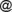# Issue:Constructions for t-conorms and t-norms on interval-valued and interval-valued intuitionistic fuzzy sets by paving

 shortcut http://ifigenia.org/wiki/issue:nifs/26/3/1-12
Title of paper: Constructions for t-conorms and t-norms on interval-valued and interval-valued intuitionistic fuzzy sets by paving
Author(s):
 Martin Kalina Department of Mathematics and Descriptive Geometry, Faculty of Civil Engineering, Slovak University of Technology Radlinskeho, 11, SK-810 05 Bratislava, Slovakia martin.kalinastuba.sk
Published in: Notes on Intuitionistic Fuzzy Sets, Volume 26 (2020), Number 3, pages 1–12
DOI: https://doi.org/10.7546/nifs.2020.26.3.1-12
Abstract: Paving is a method for constructing new operations from a given one. Kalina and Kral in 2015 showed that on the real unit interval this method can be used to construct associative, commutative and monotone operations from particular given operations (from basic ‘paving stones’). In the present paper we modify the construction method for interval-valued fuzzy sets. From given (possibly representable) t-norms and t-conorms we construct new, non-representable operations. In the last section, we modify the presented construction method for interval-valued intuitionistic fuzzy sets.
Keywords: Interval-valued fuzzy sets, Interval-valued intuitionistic fuzzy sets, Paving, t-norm, t-conorm.
AMS Classification: 03E72, 08A72.
References:
1. Atanassov, K. T. (1999). Intuitionistic Fuzzy Sets: Theory and Applications. Springer, Heidelberg.
2. Atanassov, K. T. (2020). Interval-Valued Intuitionistic Fuzzy Sets. Springer, Cham.
3. Atanassov, K., & Gargov, G. (1989). Interval valued intuitionistic fuzzy sets, Fuzzy Sets and Systems, 31 (3), 343–349.
4. Birkhoff, G. (1967). Lattice Theory. American Mathematical Society Colloquium Publishers, Providence, RI.
5. Bodjanova, S., & Kalina, M. (2017). Block-wise construction of commutative increasing monoids, Fuzzy Sets and Systems, 324, 91–99.
6. Deschrijver, G. (2008). A representation of t-norms in interval valued L-fuzzy set theory, Fuzzy Sets and Systems, 159, 1597–1618.
7. Deschrijver, G., Cornelis, C., & Kerre, E. E. (2004). On the representation of intuitionistic fuzzy t-norms and t-conorms, IEEE Trans. Fuzzy Systems, 12 (1), 45–61.
8. Goguen, J. A. (1967). L-fuzzy sets. Journal of Mathematical Analysis and Applications, 18 (1), 145–174.
9. Grabisch, M., Marichal, J. L., Mesiar, R., & Pap, E. (2009). Aggregation Functions, Cambridge University Press, Cambridge.
10. Jenei, S. (2001). Structure of left-continuous triangular norms with strong induced negations, (II) Rotation-annihilation construction, Journal of Applied Non-Classical Logic, 11, 351–366.
11. Kalina, M., & Kral, P. (2015). Construction of commutative associative operations by paving. In: Alonso, J. M., Bustince, H., Reformat M. (eds.) IFSA-EUSFLAT 2015, (Atlantis Press, Gijon, 2015), 1201–1207.
12. Klement, E. P., Mesiar, R., & Pap, E. (1999). Quasi- and pseudo-inverses of monotone functions, and the construction of t-norm, Fuzzy Sets and Systems, 104, 3–13.
13. Klement, E.P., Mesiar, R., & Pap, E. (2000). Triangular Norms. Springer, Berlin, Heidelberg.
14. Mesiarova-Zemankova, A. (2016). Ordinal sum construction for uninorms and generalized uninorms International Journal of Approximate Reasoning, 76, 1–17.
15. Schweizer B., & Sklar, A. (1983) Probabilistic Metric Spaces, North Holland, New York.
16. Zong, W., Su, Y., Liu, H. W., & De Baets, B. (2017) On the Construction of Associative, Commutative and Increasing Operations by Paving, in Torra V., Mesiar R., Baets B. (eds) Aggregation Functions in Theory and in Practice. AGOP 2017. Advances in Intelligent Systems and Computing, vol 581, Springer, Cham, 229–240.
Citations:

The list of publications, citing this article may be empty or incomplete. If you can provide relevant data, please, write on the talk page.